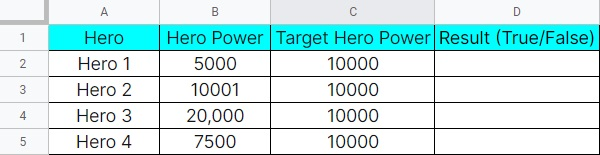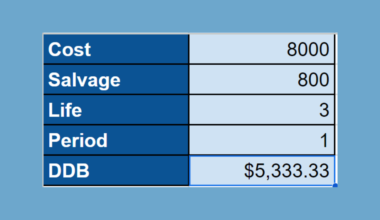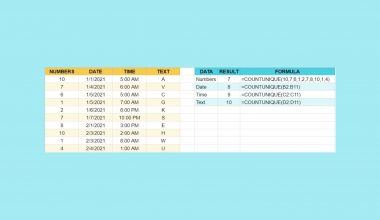# How to Use LT Function in Google Sheets

The LT function in Google Sheets is one of the comparison operators functions that yield a return value of ‘FALSE’ if the first argument is greater than the second value and ‘TRUE’ otherwise.  This function is also known as the ‘less than function.

For Math students, financial analysts, and research professionals, the `LT` function can help them to generate an answer to specific data quickly. All they need to do is to key in the value of both arguments, and the function will take care of the result.

In writing the `LT` function, you must familiarize these rules:

• The function has two arguments, value1 and value 2.
• If the first value is lesser than the second value, the function will return a result that says ‘TRUE.’
• If the second value is greater than the second value, the returning result of the function is ‘FALSE.’

Here’s one example of how to use the Google Sheets `LT` function.

A gamer needs to know which of his 100 heroes is the best pick for the championship. So, he decided to create a list of heroes and their hero power which includes the following:Granting that the gamer has around 100 heroes to choose from, how will he save time determining which hero has the best chance of winning the championship?

Google Sheets `LT` function can help the gamer in a snap. The function can generate the result based on the given values automatically. As long as the values are valid, the resulting value of the function will generate a correct result.

And though this function is not mostly used, knowing this function exists and knowing its usage and syntax is already enough. When the time comes that you need to work on a long list of numbers, you can easily and quickly work on your task.

## The Anatomy of the LT Function in Google Sheets

Here’s how you should write the `LT` function:

=LT(value1,value2)

After learning how to write it, let us learn its parts and use it to better understand how to use the function.

• = the equal sign starts the `LT` function in Google Sheets.
• LT() is our `LT` function. The returning value of the function can either be ‘True’ or ‘False’ depending on the second value, which serves as a conditional factor to the first value.
• value1 refers to the value that needs to be proven. This value must be lesser than the second value.
• value2 the second value is the conditional factor that determines the resulting value whether the first value is ‘True’ or ‘False.’

## A Real Example of Using LT Function in Google Sheets

After learning the fundamentals of Google Sheets’ `LT` function, we will now apply what we’ve learned. Then we will solve the given problem above. Since we already have an overview of the function and know how to write it, we will now apply it in Google Sheets.

Here are the data from which we will find the result.After you input all the data, we will now execute the formula using the `LT` function:

=LT(value1,value2)

Let us check the picture below and see the returning values of each hero.I will now teach on how to get the results. You can click on the link below to use the sample Google Sheet spreadsheet that I am using.

We can now practice writing the `LT` function using the step-by-step guide below if you are all set.

## How to Use LT Function in Google Sheets

1. First, you need to open a spreadsheet and input all the given data there. Here’s the data that you should input:
 Hero Hero Power Target Hero Power Hero 1 5000 10000 Hero 2 10001 10000 Hero 3 20000 10000 Hero 4 7500 10000
1. Next, after you transfer the data to the Google Sheets, we will now go ahead and start writing the function. Remember, when writing a function in Google Sheets, you must always start it with an equal ‘=’ sign.

After you key in the equal ‘=’ sign, there are some instances that a pop-up suggesting a function might show. If that happens, all you need to do is to hit ‘BACKSPACE’ on your keyboard.

1. Once the cell is clear with just an equal ‘=’ sign only, we will start adding our `LT` function. We should write it up until the open ‘(‘ parenthesis only when adding the function. This is because we will be adding the value after.2. Now, we will add value1 and value2 to the syntax. For value 1, we will substitute it with Hero Power, and for value2, we will use the Target Hero Power.Using the given data, let us substitute the value1 and value2 of the `LT` function. Once we are done substituting the values, we will complete the function with a closing ‘)‘ parenthesis.

1. Lastly, to get the result of the `LT` function, we need to hit the Enter key on our keyboard. Here is the result of Hero 1.2. After generating the first result, we can copy and paste the result into the following cells. It will automatically copy the formula and generate a result based on the value’s given numbers.

## Summary

The `LT` function is one of the basic functions in Google Sheets. However, most of us are not aware that this function exists. Now that you already have a touch base of the function and know how to write it, you can now start using this in case you need them.

Just take note of these things:

1. `LT` function is also known as Google Sheets’ “less than” comparison operator.
2. There are only two arguments in the `LT` function.
3. If the first value is bigger than the second value, the result will always be ‘FALSE.’ If it is the other way around, the returning value will be ‘TRUE.

For more How-To articles like this, you may check our site for other Google Sheets functions that you may need, and don’t forget to subscribe to our newsletter to not miss any of our uploads.Our goal this year is to create lots of rich, bite-sized tutorials for Google Sheets users like you. If you liked this one, you'd love what we are working on! Readers receive ✨ early access ✨ to new content.

##### You May Also Like## How to Use DDB Function in Google Sheets

This guide will explain how to use the DDB function in Google Sheets. When we need to calculate…## Replace Every Nth Delimiter in Google Sheets

Sometimes we get delimited data that is difficult to format. This article will explain how we can replace…## How to Get LSE Real-Time Prices in Google Sheets

Learning how to obtain prices from the LSE market in Google Sheets is useful for when you need…## How to Use COUNTUNIQUE function in Google Sheets

The COUNTUNIQUE function in Google Sheets counts the number of unique values in a dataset. It is especially…